# Day trip

A group of tourists went on a day trip. For two hours they walked at a speed of 6 km/h, for another hour they climbed a steep hill at a speed of 2 km/h, spent an hour and a half at lunch, then in an hour they inspected the castle garden at a free step at a speed of 1 m/s and finally for three hours they returned home at speed 4. km/h.
a) Calculate the total time tourists spent on the trip.
b) Calculate their average speed.

t =  8.5 h
v =  3.4824 km/h

### Step-by-step explanation:Did you find an error or inaccuracy? Feel free to write us. Thank you!Tips to related online calculators
Looking for help with calculating arithmetic mean?
Looking for a statistical calculator?
Do you want to convert velocity (speed) units?
Do you want to convert time units like minutes to seconds?

#### You need to know the following knowledge to solve this word math problem:

We encourage you to watch this tutorial video on this math problem:

## Related math problems and questions:

• Average speed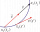The car drove on one section of the highway for half an hour at a speed of 80 km/h. Then he reduced his speed to 60km/h. It went three-quarters of an hour at a speed of 60 km/h. What was the average speed of the car?
• Pedestrian up-down hill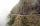Pedestrian goes for a walk first at the plane at 4 km/h, then uphill 3 km/h. Then it is in the middle of the route, turns back, and goes downhill at speed 6 km/h. The total walk was 6 hours. How many kilometers went pedestrian?
• Average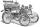What is the average speed of the car, where half of the distance covered passed at speed 66 km/h and the other half at 86 km/h.
• Average speed 4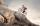Starting at home, Tony traveled uphill to the store for 45 minutes at 8 miles per hour. he then traveled back home on the same path at a speed of 24 miles per hour. What is his average speed for the entire trip?
• Cyclists and walkersA group of tourists started at 8:00 at speed 4 km/h walk. At half-past ten, another group started on a bike and caught up with a group of tourists at 10:50. What was the average speed of cyclists?
• Tourists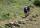From the cottage started first group of tourists at 7:00 AM at speed 4 km/h. The second started after them 21 minutes later at speed 6 km/h. How long and how many kilometers from the cottage will catch the first group?
• GrandmotherMom walked out to visit her grandmother in a neighboring village 5km away and moved at a speed of 4km/h. An hour later, father drove down the same road at an average speed of 64km/h. 1) How long will take to catch mom die? 2) What is the approximate dista
• Braking distance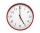The car travels at an average speed of 12 km/h and detects an obstacle 10 m in front of it. At 1 m in front of the obstacle it already runs 2 km/h. What is the braking distance? What is the required deceleration for stop in: A) 1m B) 1s?
• Scouts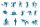Three scouts went on a three day trip. The second day went 4 km more than the first day. The third day went two times less than the first day. They went along 54 km. How many kilometers went every day together?
• Cycling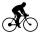Cyclist goes on a trip. First, drove 4 hours average speed 44 kilometers per hour, then slowed down and another 4 hours driving a speed 36 kilometers per hour. How many total kilometers cycling?
• Truck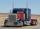Truck went for 4 hours and 30 minutes. If truck increase speed by 11 km/h, went same distance in 2 hours. Calculate this distance.
• Journey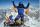The road from A to B measures 11.5 km. Firstly up the hill, then by level plane and then downhill. Tourist goes uphill at 3 km/h, on the plane 4 km/h and downhill 5 km/h. From the point, A to B went 2h 54 min back 3h 6 min. How long is the segment of the
• Two citiesThe car goes from city A to city B at an average speed of 70 km/h, back at an average speed of 50 km/h. If it goes to B and back at an average speed of 60 km/h, the whole ride would take 8 minutes less. What is the distance between cities A and B?
• Average speedThe truck drove 1/2 of the way on the highway at 80km/h. The other half of the way 20km/h. Calculate the average speed
• Two citiesThe distance between cities A and B is 132 km. At 9.00 am, the cyclist started the bike at an average speed of 24 km/h, and at 10.00 am started from the B cyclist at an average speed of 30 km/h. How long and far from A will they both meet?
• Fog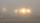The car started in fog at a speed of 30 km/h. After a 12-minute drive, the fog dissipated, and the driver drove the next 12 minutes distance, 17 km. On the last 17 km long again, the driving conditions deteriorated, and the driver drove at a speed of 51 k
• Two portsFrom port A on the river, the steamer started at an average speed of 12 km/h towards port B. Two hours later, another steamer departed from A at an average speed of 20 km/h. Both ships arrived in B at the same time. What is the distance between ports A an